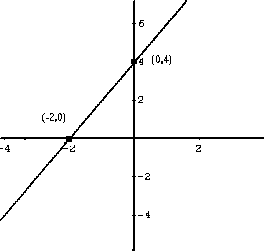# Graphing Linear Functions

Graph a linear function: a step by step tutorial with examples and detailed solutions. Free graph paper is available.

## Linear Functions

Any function of the form f (x) = m x + b, where m is not equal to 0 is called a linear function. The domain of this function is the set of all real numbers. The range of f is the set of all real numbers. The graph of f is a line with slope m and y intercept b.
Note: A function f (x) = b, where b is a constant real number is called a constant function. Its graph is a horizontal line at y = b.

### Example 1

Graph the linear function f given by
f (x) = 2 x + 4

Solution to Example 1

• You need only two points to graph a linear function. These points may be chosen as the x and y intercepts of the graph for example.
• Determine the x intercept, set f(x) = 0 and solve for x.

2 x + 4 = 0

x = - 2

• Determine the y intercept, set x = 0 to find f(0).

f(0) = 4

• The graph of the above function is a line passing through the points (- 2 , 0) and (0 , 4) as shown below.Matched Problem
Graph the linear function f given by
f (x) = x + 3

### Example 2

Graph the linear function f given by
f (x) = - (1 / 3) x - 1 / 2

Solution to Example 2

• Determine the x intercept, set f(x) = 0 and solve for x.

-(1 / 3) x - 1 / 2 = 0
x = - 3 / 2

• Determine the y intercept, set x = 0 to find f(0).
f(0) = -1 / 2

• The graph of the above function is a line passing through the points (-3 / 2 , 0) and (0 , -1 / 2) as shown below.

•Matched Problem 2
Graph the linear function f given by
f (x) = - x / 5 + 1 / 3

## More References and Links to Graphing and Graphs of Functions

Graphing Functions
Solver to Analyze and Graph a Linear Function